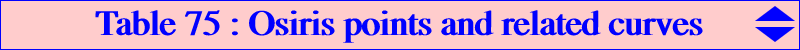Osiris points are defined by César Lozada in the preamble just before X(40328) in ETC which is copied below. Let ABC be a triangle, P a point and Q the isotomic conjugate of P. Denote by A' the centroid of the quadrangle BCPQ and define B' and C' cyclically. Then AA', BB', CC' concur at a point O(P) = O(Q), here named the Osiris point of P (and Q). For P = x : y : z (barycentrics), O(P) = (y^2+5*y*z+z^2)*x+2*(y+z)*(x^2+y*z) : : , and a list of pairs {P, O(P)} is given in ETC. *** The (3rd degree) mapping P → O(P) • has five singular points namely A, B, C and the infinite points St1, St2 of the Steiner ellipse. • has four fixed points namely G and A1, B1, C1 where A1 is the image of A under the homothety h(G, – 5/4) and B1, C1 are defined likewise. • transforms every cK0(#X2, R at infinity) into a line which is the conjugated diameter of the line GR in the Steiner ellipse. For instance, the cubics K185, K296, K953 are transformed into the lines passing through G and X(6), X(1), X3) respectively. *** Now, given a fixed point P = p : q : r ≠ G, the locus of the variable point M such that P, M, O(M) are collinear is the union of the line at infinity and a circum-cubic Os(P), here named the Osiris cubic of P, which is always a K0 (no term in x y z). It must pass through G (with tangent passing through P) and the fixed points A1, B1, C1. The equation of Os(P) is : ∑ p (y - z) [2x (y + z) + y z] = 0. Denote by P1 = aaP the anticomplement of the anticomplement of P and by P2 = taaP its isotomic conjugate. P2 lies on Os(P). Os(P) meets the line at infinity at the same points as pK(G, P1). The six other finite common points lie on the circum-conic passing through G(counted twice) and P2. This conic is the isotomic transform of the line GP hence it contains tP and many other simple points. Os(P) meets the Steiner ellipse at the same points as pK(G, P) namely A, B, C an three other (real or not) points S1, S2, S3. The two cubics have three more common points which are G (twice) and P. Special Osiris cubics • Os(P) cannot be a pK unless it decomposes into the medians of ABC when P = G. When P is another point on a median, Os(P) splits into this same median and a (possibly decomposed) conic. • Os(P) is a nK0 when P lies on the Tucker cubic obtained for λ = 2/9 without any known center on the curve. • Os(P) is circular if and only if P = X(625) giving the cubic K1167. • Os(P) is equilateral if and only if P = X(40344) giving the cubic K1168. • Os(P) meets the Steiner ellipse at the vertices S1, S2, S3 of an equilateral triangle when P = X(11055). *** The following table shows a selection of remarkable Os(P) computed by Peter Moses.P X(i) on Os(P) for i = remark 625 2, 67, 524, 625, 40341, 40342, 40343 K1167, circular 40344 2, 11058, 40344 K1168, equilateral 620 2, 523, 620, 3413, 3414, 36955 3834 2, 80, 519, 3632, 3834 4395 2, 519, 3244, 4395, 39697 7227 2, 321, 553, 4681, 7227 17067 2, 519, 1120, 17067, 20050 17768 2, 9436, 11599, 17768, 35141